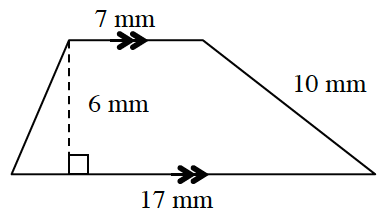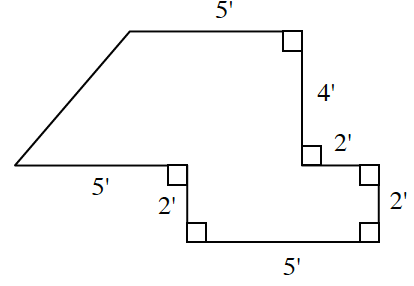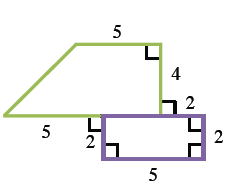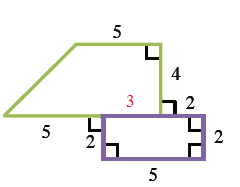### Home > GB8I > Chapter 10 Unit 11 > Lesson INT1: 10.1.2 > Problem10-38

10-38.

Find the area and perimeter of each polygon below. Show all work.

1.1.Divide the figure into two separate shapes.
Afterwards, find the area for each shape and then add them together.

The piece shared between the trapezoid and the rectangle is $5 - 2 = 3$ because the red rectangle has side $5$. This makes the base of the trapezoid $8$.

Area of Shape = Area of Trapezoid + Area of Rectangle

$\frac{1}{2}\left(\textit{b}_1+\textit{b}_2\right)+\textit{bh}$

$\frac{1}{2}([5+3]+5)4+2\cdot 5$

Total area $= 36\text{ units}^2$